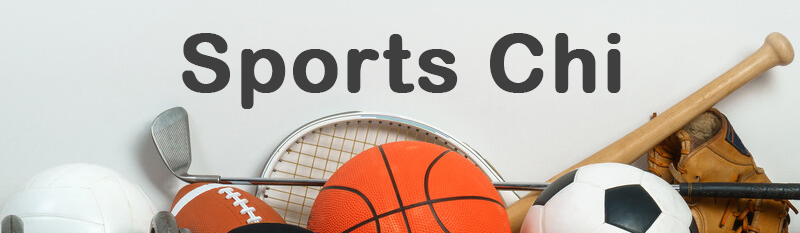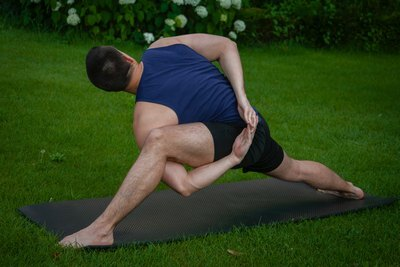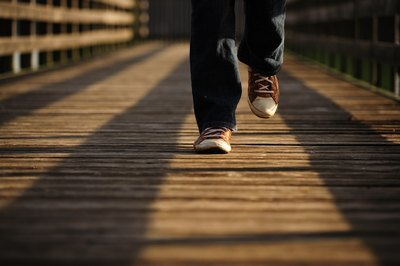Like   Tweet   Pin   +1   in/* styles */ ... Tips and resources on performance and wellbeing   ||   Issue #3, May 2021
 table div table+table+table div table{width:100%;padding:0}table div table+table+table div table img{width:96.23%;padding:0;float:none}table div table+table+table div table td{width:100%;padding:0 1.88% 18px}/* styles */Dear Reader,

Almost, but not quite there. I tried to publish the April edition of Sports Chi but ran out of time. I got caught up in trying to earn a crust - marketing, organising and delivering Chair Chi workshops.

But I'm back on track. In this issue there’s an article on muscle pain relief, a Tai Chi Tip on balance, a motivational clip of an autistic basketball player, an amusing quote, a coaching tip and a Lao Tzu quote.

Regards
Chris Bennett
Chris Chi

 table div table+table+table+table+table div table{width:100%;padding:0}table div table+table+table+table+table div table img{width:96.23%;padding:0;float:none}table div table+table+table+table+table div table td{width:100%;padding:0 1.88% 18px}/* styles */## Muscle Pain Relief

 /* styles */Here’s a handy article regarding muscle pain relief by Patricia Carswell.

She covers eight techniques which include: ‘have a massage; foam rolling; hot and cold treatment; stretch it out; eat your way out of pain; chill out; sleep tight; and get back in the gym’.

I do seven out of eight of her suggestions, on a regular basis, when it comes to muscle soreness due to training. The one tip I don’t do is eat my way out of pain - that's a new one to me.

Image courtesy of Adél Grőber via Unsplash

 table div table+table+table+table+table+table+table+table+table div table{width:100%;padding:0}table div table+table+table+table+table+table+table+table+table div table img{width:96.23%;padding:0;float:none}table div table+table+table+table+table+table+table+table+table div table td{width:100%;padding:0 1.88% 18px}/* styles */## Tai Chi Tip: BalanceDirect your mind to sink your weight into the supporting leg, as you step forward or backward.

Do this slowly so you can get a good workout.

Image courtesy of Frank Busch via Unsplash

 table div table+table+table+table+table+table+table+table+table+table+table+table div table{width:100%;padding:0}table div table+table+table+table+table+table+table+table+table+table+table+table div table img{width:96.23%;padding:0;float:none}table div table+table+table+table+table+table+table+table+table+table+table+table div table td{width:100%;padding:0 1.88% 18px}/* styles */## Jason's Barrage of ShotsHere's an inspirational clip of austic basketball player Jason McElwain.

Credit to Jason for his wonderful achievement and also to his coach, who gave him the opportunity to perform his incredible feat.

 table div table+table+table+table+table+table+table+table+table+table+table+table+table+table+table div table{width:100%;padding:0}table div table+table+table+table+table+table+table+table+table+table+table+table+table+table+table div table img{width:96.23%;padding:0;float:none}table div table+table+table+table+table+table+table+table+table+table+table+table+table+table+table div table td{width:100%;padding:0 1.88% 18px}/* styles */## Ehh!I couldn't resist sharing this quote from Philosophy Matters. It's from a lesser known ancient Greek philosopher.

'ehh, good enough'
Mediocrates

Image courtesy of Eran Menashri via Unsplash

 table div table+table+table+table+table+table+table+table+table+table+table+table+table+table+table+table+table+table div table{width:100%;padding:0}table div table+table+table+table+table+table+table+table+table+table+table+table+table+table+table+table+table+table div table img{width:96.23%;padding:0;float:none}table div table+table+table+table+table+table+table+table+table+table+table+table+table+table+table+table+table+table div table td{width:100%;padding:0 1.88% 18px}/* styles */## Coaches' Corner

 /* styles */ Set an example for your players by looking after your own psychological, physical and emotional health.
 table div table+table+table+table+table+table+table+table+table+table+table+table+table+table+table+table+table+table+table+table+table div table{width:100%;padding:0}table div table+table+table+table+table+table+table+table+table+table+table+table+table+table+table+table+table+table+table+table+table div table img{width:96.23%;padding:0;float:none}table div table+table+table+table+table+table+table+table+table+table+table+table+table+table+table+table+table+table+table+table+table div table td{width:100%;padding:0 1.88% 18px}/* styles */table div table+table+table+table+table+table+table+table+table+table+table+table+table+table+table+table+table+table+table+table+table+table+table div table{width:100%;padding:0}table div table+table+table+table+table+table+table+table+table+table+table+table+table+table+table+table+table+table+table+table+table+table+table div table img{width:96.23%;padding:0;float:none}table div table+table+table+table+table+table+table+table+table+table+table+table+table+table+table+table+table+table+table+table+table+table+table div table td{width:100%;padding:0 1.88% 18px}/* styles *//* styles */
 table div table+table+table+table+table+table+table+table+table+table+table+table+table+table+table+table+table+table+table+table+table+table+table+table+table div table{width:100%;padding:0}table div table+table+table+table+table+table+table+table+table+table+table+table+table+table+table+table+table+table+table+table+table+table+table+table+table div table img{width:96.23%;padding:0;float:none}table div table+table+table+table+table+table+table+table+table+table+table+table+table+table+table+table+table+table+table+table+table+table+table+table+table div table td{width:100%;padding:0 1.88% 18px}/* styles *//* styles */